VP 110: Bytes and Files (25 pts)

What You Need

Any computer with Python 3.

Purpose

Learn about the Python 3 data types: byte, int, and string.

Integers

Execute this command to open Python 3 in interactive mode:
python3
Now enter these commands, which are immediately executed.
i = 1
print(i, type(i))
j = i + 1
print(j, type(j))
As shown below, i and j are 'int' objects: integers, so addition works.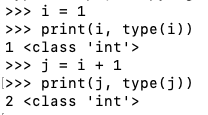Bytes

Execute these commands:
a = b'\x01'
print(a, type(a))
b = a + 1
As shown below, a is a "bytes" object, and addition is not allowed.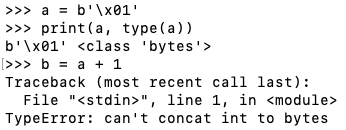One way to convert bytes to integers is to select a specific byte.

Execute these commands:

b = a
print(b, type(b))
c = b + 1
print(c, type(c))
As shown below, b is a "int" object, and addition works.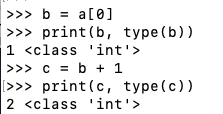So, to add one to a byte object, execute these commands:
a = b'\x01'
print(a, type(a))
b = a
print(b, type(b))
c = b + 1
print(c, type(c))
d = bytes([c])
print(d, type(d))
As shown below, converting the "bytes" object to an "int" object, performing the addition, and converting it back to "bytes" works.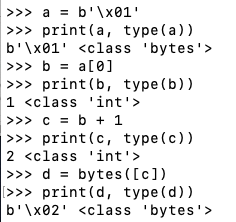To exit interactive mode, execute this command:
exit()

Files

In a text editor, create a file named infile containing this text:
HELLO
In a text editor, make this program:
with open("infile", "rb") as f:
while byte != b"":
print(byte)
As shown below, this program reads the file one byte at a time.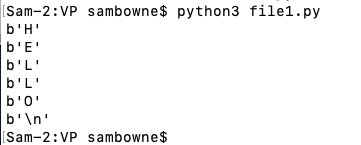In a text editor, make this program:
with open("infile", "rb") as f:
with open("outfile", "wb") as g:
while byte != b"":
print(byte)
g.write(byte)
As shown below, this program copies infile to outfile.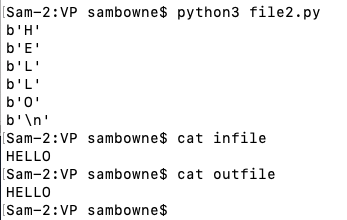Flag VP 110.1: Eights (5)

https://samsclass.info/124/proj14/VP110-1
sudo apt update
sudo apt install wget -y
wget https://samsclass.info/124/proj14/VP110-1
Count the number of '\x08' bytes. That's the flag.

Flag VP 110.2: Adding Files (10)

https://samsclass.info/124/proj14/VP110-2a
https://samsclass.info/124/proj14/VP110-2b
Add the bytes in the two files together to construct the flag. For example, if the first file contains b'\x40' and the second contains b'\x01', the result is b'\x41', or "A".